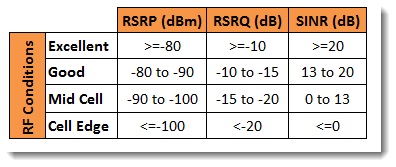# RSRQ (Reference Signal Received Quality)

RSRQ is th Reference Signal Received Quality and it can be defined using following.

As per the definition of RSSI, It is wide band power including signal power from serving cell, co-channel neighbour cell, interference from other cell interference and noise, in similar word one can say that RSRQ define the purity of Reference Signal (RS) across the system bandwidth. RSRQ is a calculated value from RSRP and RSSI is measure of signal and interference. As RSRQ is a ration of two signal powers with same  same unit i.e. dBm so RSRQ uses dB as a measurement unit.

Similar to RSRP , UE reported an integer value to eNodeB and its range is from 0 to 34. 3GPP has provided a table shown below, by using this table the integer value can be translated to a range of RSRP value in dB.

The best value of RSRP is about -3 dB and worst value can be -19.5dB.

Reported RSRQ (Integer) From RSRQ Value (dB) To RSRQ Value (dB)
00  – 19.5 dB
01 – 19.5 dB – 19.0 dB
02 – 19 dB -18.5 dB
03 -18.5 dB – 18.0 dB
04 – 18.0 dB – 17.5 dB
05 – 17.5 dB – 17.0 dB
06 – 17.0 dB – 16.5 dB
07 – 16.5 dB – 16.0 dB
08 – 16.0 dB – 15.5 dB
09 – 15.5 dB – 15.0 dB
10 – 15.0 dB – 14.5 dB
11 – 14.5 dB – 14.0 dB
12 – 14.0 dB – 13.5 dB
13 – 13.5 dB – 13.0 dB
14 – 13.0 dB – 12.5.0 dB
15 – 12.5 dB – 12.0 dB
16 – 12.0 dB – 11.5 dB
17 -11.5 dB – 11.0 dB
18 – 11.0 dB -10.5 dB
19 – 10.5 dB – 10.0 dB
20 – 10.0 dB – 9.5 dB
21 – 9.5 dB – 9.0 dB
22 – 9.0 dB – 8.5 dB
23 – 8.5 dB – 8.0 dB
23 – 8.0 dB – 7.5 dB
25 – 7.5 dB – 7.0 dB
26 – 7.0 dB – 6.5 dB
27 – 6.5 dB – 6.0 dB
28 – 6.0 dB – 5.5 dB
29 – 5.5 dB – 5.0 dB
30 – 5.0 dB – 4.5 dB
31 – 4.5 dB – 4.0 dB
32 – 4.0 dB – 3.5 dB
33 – 3.5 dB – 3.0 dB
34 – 3.0 dB

So, when there is no traffic, and assuming only the reference symbols are transmitted (there are 2 of them within the same symbol of a resource block) from a single Tx antenna then the RSSI is generated by only the 2 reference symbols so the result becomes

• RSRQ = N / 2N = -3 dB for 1Tx
• RSRQ = -6 dB for 2Tx

If all resource elements are active and are transmitted with equal power, then

• RSRQ = N / 12N = -10.8 dB for 1Tx
• RSRQ = N / 20N = -13 dB for 2Tx

(because RSRP is measured over 1 resource element and RSSI per resource block is measured over 12 resource elements).

Related Posts: